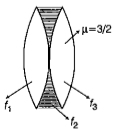# NEET Physics Ray Optics and Optical Instruments Questions SolvedNEET - 2016

Two identical glass $\left({\mu }_{g}=3/2\right)$ equi-convex lenses of focal length $f$ each are kept in contact. The space between the two lenses is filled with water $\left({\mu }_{w}=4/3\right)$. The focal length of the combination is

(a)  $f/3$             (b) $f$

(c) $\frac{4f}{3}$               (d) $\frac{3f}{4}$

(d) consider the situation shown is figure. Let radius of curvature of lens surfaces is R. The combination is equivalent to three lenses in contact.Now$\frac{1}{{f}_{1}}=\frac{1}{{f}_{3}}=\frac{1}{f}=\left(\mu -1\right)\left(\frac{1}{R}-\frac{1}{-R}\right)=\left(\mu -1\right)\left(\frac{2}{R}\right)⇒R=2f\left(\mu -1\right)$

Difficulty Level:

• 13%
• 27%
• 28%
• 33%
Crack NEET with Online Course - Free Trial (Offer Valid Till September 21, 2019)